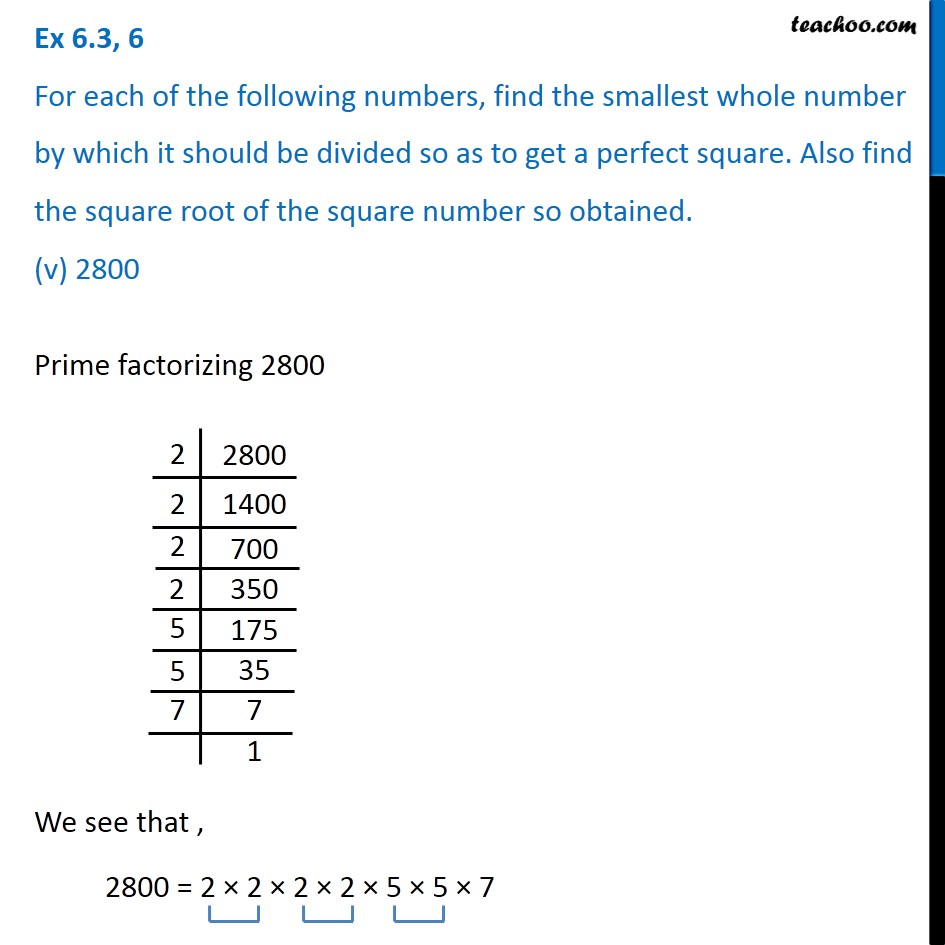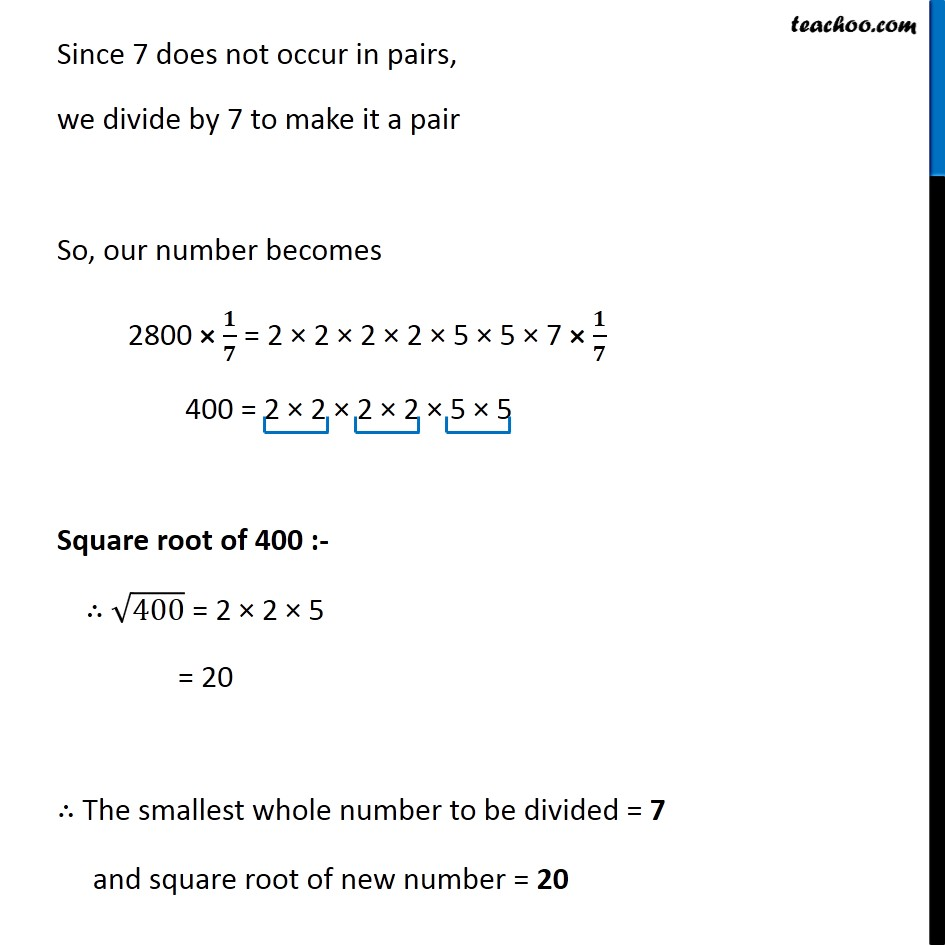Ex 6.3

Chapter 6 Class 8 Squares and Square Roots
Serial order wise### Transcript

Ex 6.3, 6 For each of the following numbers, find the smallest whole number by which it should be divided so as to get a perfect square. Also find the square root of the square number so obtained. (v) 2800Prime factorizing 2800 We see that , 2800 = 2 × 2 × 2 × 2 × 5 × 5 × 7 Since 7 does not occur in pairs, we divide by 7 to make it a pair So, our number becomes 2800 × 𝟏/𝟕 = 2 × 2 × 2 × 2 × 5 × 5 × 7 × 𝟏/𝟕 400 = 2 × 2 × 2 × 2 × 5 × 5 Square root of 400 :- ∴ √400 = 2 × 2 × 5 = 20 ∴ The smallest whole number to be divided = 7 and square root of new number = 20